Custom Search

## Friday, July 25, 2008

### MODERN PHYSICS

VTU Model Question Paper
1.a. I If the momentum of a particle is increased to four times, the de-Broglie wavelength is
A) become twice B) become for times C) become one-fourth D) become half
II Blackbody radiation spectrum, maximum intensity is shifting towards
A) shorter wavelength B) longer wavelength C) no change D) none of these
III Group velocity of wave is equal to
A) V phase B) V particle C) Velocity of light D) none of these
IV de-Broglie wavelength of an electron accelerated by a potential of 60 V is
A) 1.85 Å B) 1.58 Å C) 1.589 Å D) 1.57 Å
b. Describe Davisson-Germer experiment to prove the dual nature of matter waves.
c. Explain phase velocity and Group velocity. Derive de-broglie wavelength using Group velocity. (4+8+8)

Dec 08/ Jan 09
1 a. 1) The de-Broglie wavelength associated with an electron of mass m and accelerated by a potential V is

2) Davisson and Germer were the first to demonstrate :
i) The straight line propagation of light ii) The diffraction of photons
iii) The effective mass of electron iv) None of these
3) Electrons behaves as waves because they can be :
i) Deflected by an electric field ii) Diffracted by a crystal
iii) Deflected by magnetic field iv) They ionize a gas
4) In Davisson-Germer experiment, the hump is most prominent when the electron is accelerated by
i) 34 volts ii) 54 volts iii) 60 volts iv) 80 volts (04 Marks)
b. Define phase velocity and group velocity. Show that group velocity is same as particle velocity. (08 Marks)
c. Derive de-Broglie wavelength using Group velocity. (04 Marks)
d. Compare the energy of a photon with that of a neutron when both are associated with wavelength of 1 Å. Given that mass of neutron is 1.678 × 10 -27 kg. (04 Marks)

June-July 2009
1 a. i) An electron and a proton are accelerated through same potential. The ratio of de-Broglie wavelength e/p is

ii) Wavefunction associated with a material particle is
A) Single valued B) Finite C) Continuous D) ALL the above
iii) In a blackbody radiation spectrum, the maximum energy peaks shift towards the shorter wavelength side with the increase in temperature. This confirms
A) Stefan’s law B) Wein’s law C) Rayleigh-Jean’s law
D) Planck’s law
iv) The group velocity of the particle is 3×106 m/s, whose phase velocity is
A) 6.06×106 m/s B) 3×1010 m/s C) 3 nm/s D) 1.5×1010 m/s (04 Marks)
b. Describe Davisson and Germer experiment for confirmation of deBroglie hypothesis.
(08 Marks)
c. Explain phase and group velocity. Calculate the de-Broglie wavelength of a bullet of mass 5 gm moving with a velocity 20 km/hr. (08 Marks)

Dec.09/Jan.10
1 a. i) Wien’s law is deduced from Planck’s radiation formula under the condition of
A) Very small wavelength and temperature B) Large wavelength and temperature
C) Small wavelength and high temperature D) Large wavelength and small temperature
ii) The Compton wavelength is given by
A) h/m0C2 B) h2/m0C2 C) h/m0C D) h2/2m0C
iii) Which of the following relations can be used to determine de-Broglie wavelength associated with a particle?

iv) If the group velocity of a particle is 3×106 m/s, its phase velocity is
A) 100 m/s B) 3×106 m/s C) 3×108 m/s D) 3×1010 m/s
(04 Marks)
b. What is Planck’s radiation law? Show how Wien’s law and Rayleigh-Jean’s law can be derived from it. (06 Marks)
c. Define group velocity. Derive relation between group velocity and phase velocity.
(06 Marks)
d. A fast moving neutron is found to be have a associated de-Broglie wavelength 2Å. Find its kinetic energy and group velocity of the de-Broglie waves. (04 Marks)

May/June 2010
1 a. i) In a blackbody radiation spectrum, the Wien’s distribution law is applicable only for
A) Longer wavelength B) Shorter wavelength C) Entire wavelength
D) None of these
ii) The de-Broglie wavelength associated with an electron of mass m and accelerated by a potential V is

iii) Electrons behaves as a wave because they can be
A) Diffracted by a crystal B) Deflected by magnetic field
C) Deflected by electric field D) Ionise a gas
iv) If the group velocity of de-Broglie wave is 4×108 m/sec, its phase velocity is
A) 12×108 m/sec B) 2.25×108 m/sec C) 5.33×108 m/sec D) 1.33×108 m/sec
(04 Marks)
b. Explain duality of matter waves. (04 Marks)
c. Define phase velocity and group velocity. Show that group velocity is equal to particle velocity. (08 Marks)
d. Calculate the momentum of the particle and de-Broglie wavelength associated with an electron with a kinetic energy of 1.5 keV. (04 Marks)
January 2011
i) Green light incident on a surface releases photoelectrons from the surface. If now blue light is incident on the same surface, the velocity of electrons
A) increases B) decreases C) remains same D) becomes zero
ii) Rayleigh-Jean’s theory of radiations agree with experimental results for
A) all wavelengths B) shorter wavelengths only C) longer wavelengths only
D) middle order wavelengths only
iii) The de-Broglie wavelength of an electron accelerated to a potential difference of 100 volts is
A) 1.2Å B) 10Å C) 100Å D) 12Å
iv) The wave nature associated with electrons in motion was verified by
A) photoelectric effect B) Compton effect C) diffraction by crystals
D) Raman effect (04 Marks)
b. State and explain de-Broglie’s hypothesis. (04 Marks)
c. Define phase velocity and group velocity. Obtain the relation between group velocity and particle velocity. Obtain the expression for de-Broglie wavelength using group velocity. (08 Marks)
d. Find the kinetic energy and group velocity of an electron with de-Broglie wavelength of 0.2 nm.

An object which absorbs all radiation that is incident on it is called a blackbody. A perfect blackbody is an assumption and we can have objects that are only close to a blackbody. A blackbody, on heating, can emit all radiations it has absorbed and is called blackbody radiation. Figure shows the energy distribution curves in which energy density E (energy emitted by the blackbody per unit area of the surface) is plotted as a function of wavelength at different temperatures of the blackbody.The important points that can be noted down from these curves are
1. All curves shows a peak suggesting that the emitted intensity is maximum at a particular wavelength.
2. An increase in temperature results in an increase in the energy emitted.3 .As the temperature increases, the peak shifts to lower wavelength.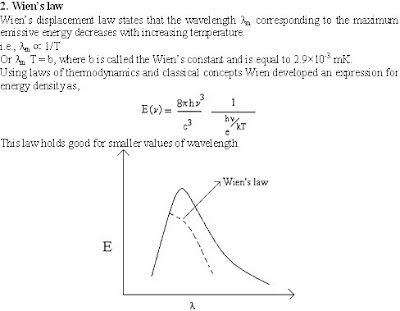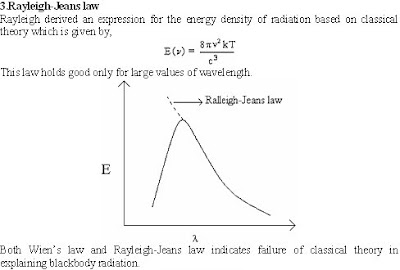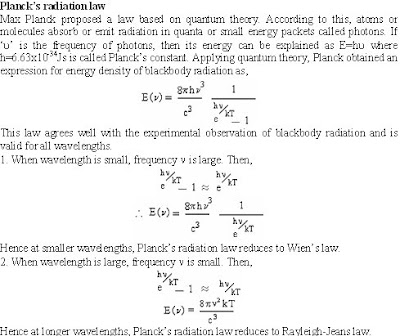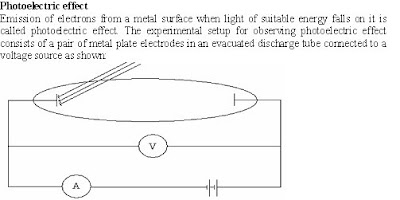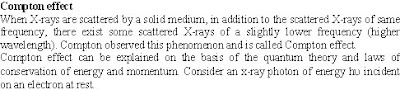Davisson-Germer experiment
The electron diffraction experimental setup used by Davisson and Germer to verify de-Broglie’s hypothesis is as shown: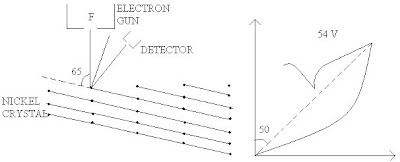The filament F is heated to produce electrons via thermionic emission. These electrons are passed through a narrow aperture forming a fine beam of accelerated electrons. The electron beam was then made to incident on a single crystalline sample of Nickel. The electrons scattered at different angles were counted using a detector. The experiment was repeated by recording the scattered electron intensities at various positions of the detector.
A sharp maximum occurred in electron density at an angle of 500 with the incident beam for an accelerating potential of 54 V. The angle of incidence corresponding to this is 250 and from figure, glancing angle (angle of diffraction) is 650. From X-ray diffraction experiment, spacing of the planes responsible for diffraction was found to be 0.091 nm.
Assuming first order diffraction, Bragg’s law can be written as,
l = 2d sinq = 2×0.091×10-9×sin65 = 0.165 nm.
By de-Broglie relation,
l = h/(2meV)1/2 = (6.626×10-34) / (2×9.1×10-31×1.6×10-19×54)1/2 = 0.167 nm.
Thus Davisson and Germer experiment directly verifies the de-Broglie hypothesis.

Characteristic properties of matter waves
1. Matter waves are associated with moving particle.
2. Wavelength of matter waves is inversely proportional to the velocity with which the particle is moving (l = h/mv). Hence a particle at rest has an infinite wavelength.
3. Wavelength of matter waves is inversely proportional to the mass of the particle. Hence wavelike behaviour of heavier bodies is not very evident whereas wave nature of subatomic particles could be observed experimentally.
4. Wavefunction is used to define a matter wave which is related to the probability of finding a particle at any place at any instant.
5. Matter waves are represented by a wave packet made up of a group of waves of slightly differing wavelengths. Hence we talk of group velocity of matter waves rather than the phase velocity (velocity of a single wave). The group velocity can be shown to be equal to the particle velocity.